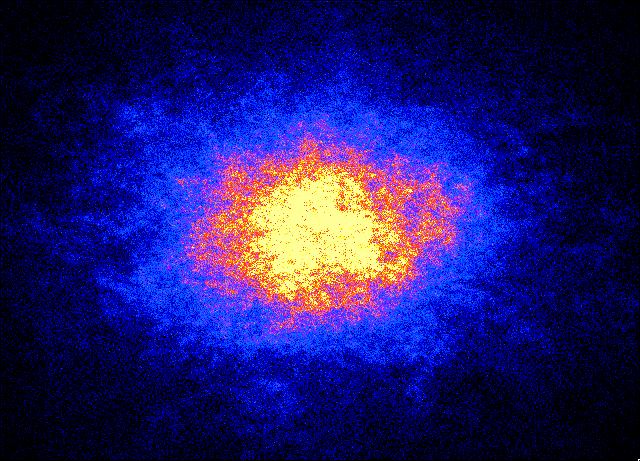## OrdinaryDifferential Equations II

Winter 2015
MWF 9:00-9:50
CAB 457University of Alberta
Mathematical and Statistical Sciences
Dr. Thomas Hillen
492-3395, thillen@ualberta.ca

Cab 575

Summary:

In this course we will study asymptotics of ordinary differential equations and boundary value problems. The Poincare-Bendixson theory has been covered in Math 524. We cover the theory of dynamical systems and differential equations in Banach spaces. The concepts of stability and bifurcations can be generalized from ODEs to PDEs. We will  systematically derive a theory of finite dimensional  compact global attractors, and we will investigate two examples in detail: the Navier-Stokes equations and reaction-diffusion equations.

### Texts:

• J.C. Robinson.  Infinite-Dimensional Dynamical Systems. Cambridge University Press, 2001.

• R. Temam.  Infinite-Dimensional Dynamical Systems in Mechanics and Physics. Springer, 1988
• O.A. Ladyzhenskaya, Attractors for Semigroups and Evolution Equations, Cambridge 1991.
• M.W. Hirsh, S. Smale.  Differential Equations, Dynamical Systems, and Linear Algebra. Academic Press, 1974.
• L. Perko. Differential Equations and Dynamical Systems. Springer, 3rd ed., 2001
• A.V. Babin, M.I. Vishik, Attractors of Evolution Equations. North-Holland, 1992

6 Homework Assignments of equal  weight = 100%

### Contact:

Dr. Thomas Hillen, 492-3395, thillen@ualberta.ca
office hours: after calss 10:00-10:45, or by appointment,   CAB 575.

Lecture Notes

Assignments due at 9 AM in class:
1. Assignment 1 (Jan 16, 2015)
2. Assignment 2 (Jan 30, 2015)
3. Assignment 3 (Wednesday, Feb 25, 2015)
4. Assignment 4 (Mar 13, 2015)
5. Assignment 5 (Mar 27, 2015)
6. Assignment 6 (Apr 10, 2015)
1. Introduction

We skip the following sections (1.5) -(1.7) since they were covered in Math 524:

2. Some Functional Analysis
3. Reaction-Diffusion Equations
4. The Navier Stokes Equation
5. Global Attractors
6. Global Attractor for Reaction-Diffusion Equations in 1-D
7. Global Attractor for Navier-Stokes Equations in 2-D
8. Finite Dimensional Attractors